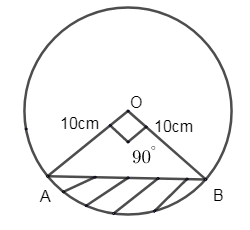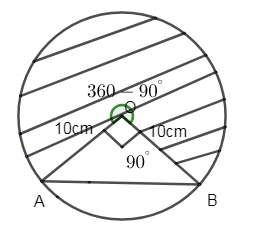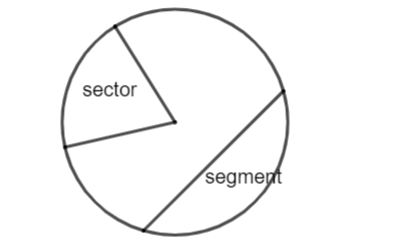Courses
Courses for Kids
Free study material
Free LIVE classes
MoreLIVE
Join Vedantu’s FREE Mastercalss

# A chord of a circle of radius 10cm subtends a right angle at the center. Find the area of the corresponding (i) Minor segment (ii) Major sector (use$\pi =3.14$).Verified
333.9k+ views
Hint: Find the area of the minor segment by subtracting the area of the triangle formed by chord from the area of minor sector. Where$\theta ={{90}^{\circ }}$ for the minor sector and for finding the area of the major sector, the angle becomes $\left( 360-\theta \right)$.

(i) Minor segment
Given that the radius of the circle is 10cm.
It’s center is marked as O.
OA and OB are the radii of the triangle.$\therefore$OA = OB = 10cm.
Here AB refers to the chord of the circle.
Given that the chord subtends a right angle at the center of the circle.
$\therefore \theta ={{90}^{\circ }}$
We need to find the area of the minor segment AOB.
Area is given by the formula, $\dfrac{\theta }{360}\times \pi {{r}^{2}}$.
$\therefore$ Area of minor sector OAPB$=\dfrac{\theta }{360}\times \pi {{r}^{2}}=\dfrac{90}{360}\times 3.14\times {{\left( 10 \right)}^{2}}$
\begin{align} & =\dfrac{1}{4}\times 3.14\times 100 \\ & =\dfrac{314}{4}=78.5c{{m}^{2}} \\ \end{align}
Now we will find area of triangle AOB,
Area of triangle AOB = $\dfrac{1}{2} \times$ Base $\times$ Height
Area of triangle AOB = $\dfrac{1}{2} \times$ OB $\times$ AO
Area of triangle AOB = $\dfrac{1}{2} \times$ 10 $\times$ 10
Area of triangle AOB = 50 $\text{cm}^2$
So now,
Area of minor segment = Area of Minor sector - Area of triangle AOB
Area of minor segment = 78.5 - 50
Area of minor segment = 28.5 $\text{cm}^2$

(ii) Major sectorAngle =$360-\theta =360-{{90}^{\circ }}$ of the shaded portion.
Area of major sector is given by formula,
\begin{align} & =\dfrac{360-\theta }{360}\times \pi {{r}^{2}} \\ & =\dfrac{360-90}{360}\times 3.14\times {{10}^{2}}=\dfrac{270}{360}\times 314 \\ \end{align}
$=\dfrac{3}{4}\times 314=235.5c{{m}^{2}}$.
$\therefore$ Area of minor sector =28.5$c{{m}^{2}}$.
Area of major sector = 235.5$c{{m}^{2}}$.

So, Area of major sector = 235.5$c{{m}^{2}}$.

Note: The sector and segment of a circle are entirely different.The sector can be called a “pizza” slice and the segment which is cut from the circle by a “chord”.
Remember to take the value of $\theta$ in the major sector as $\left( 360-\theta \right)$ and not${{90}^{\circ }}$. As we are finding the shaded region, we should that angle value.
Last updated date: 21st Sep 2023
Total views: 333.9k
Views today: 8.33k